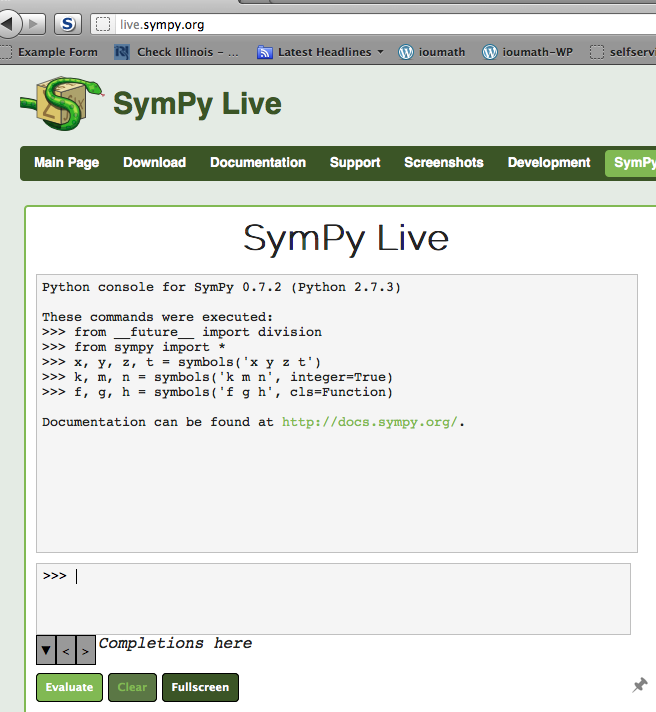Arithmetic with Python
24jun13

\begin{document}
\maketitle\section{Introduction}
Python is a popular computer language which has a useful feature, enabling you
to do arithmetic. Every calculator can do that! True, but Python is \textit{extensible}. That means that you can build your own algorithms. It is as if you
could add a new button to your calculator to do something new, easily.

MORE on the introduction.

\textbf{If you know Python and use it on your computer, skip to the section on solving Diophantine Equations}

\section{SymPy Live}
Many computer operating systems come with Python built in. If yours does not
for a start, we can use a webservice called
SymPy Live .

This is a webservice, much like our texWins, where a full Python program is
is not as nice, or as versatile as using the "real thing" on your computer.
But it works well enough for our purposes.

\section{SymPy Lessons}
First Lesson on Long Division

MORE on how to use SymPy Live
\section{On Solving Diophantine Equations}

The lesson that ends this discuss is on
Solving Diophantine Equations
using the Python functions in Jim Carlson's essay, and also how
to solve these equations by hand. You will need to understand Euclid's
algorithm for it.

To use Carlson's functions you will need to import or import * the
\item \texttt{ldv(a,b)} prints the quotient and remainder of long division of the dividend $a$ by the divisor $b$.
\item \texttt{euc(a,b)} prints the greatest commond divisor $gcd(a,b)$.
\item \texttt{isolve(a,b,c)} prints one solution to $ax+by=c$.
\end{document}